Next: 4. Pitches, Intervals, and Up: course-notes Previous: 2. Sinusoids   Index

Subsections

# 3. Spectra

As we saw in Section 2.1, the three fundamental operations on signals have the property that if their inputs are sinusoidal (and if there is more than one input, if they share the same frequency), then the output is also a sinusoid at the same frequency. The only things that might change are the sinusoid's amplitude and/or initial phase.

It's also true of many physical objects or systems that, if you apply a sinusoidal force at one point and measure the resulting motion at another, you'll also get a sinusoid of the same frequency, and that the output amplitude is proportional to the input amplitude.

This is at least one reason (perhaps the primary reason) why sinusoids are important: if you can describe a signal in terms of sinusoids, and if you know that some operation or other acts on sinusoids only by changing their amplitudes and phases, then you might be able to find what the system will do to your overall signal.

In general, a description of the makeup of a signal as sum of sinusoids is called a spectrum, and is the subject of this chapter. In the next section we'll try to make a more precise description of two possible definitions for the spectrum of a signal. In section 3.2 we'll consider how systems that preserve sinusoids--specifically, filters--affect the spectra of signals they are applied to.

The spectrum of a signal can be related to what we hear as the signal's timbre (a catch-all term that just means what a thing sounds like), and so operations (such as filters) that have predictable effects on signals' spectra can be very useful in synthesizing and processing sounds. In section 3.3 we'll take up one example of this, called subtractive synthesis.

# 3.1 Definitions and Examples

In the field of acoustics, one sees at least two important types of spectra: real ones (usually obtained by making measurements on a digital recording) and idealized ones (that might arise, for example, in theoretical analyses of various systems or be specified out of thin air for compositional or other reasons). In either case, a spectrum can be thought of as a graph whose horizontal axis shows frequency and whose vertical axis shows the relative strength of a signal (or other thing) at each frequency.

Real, measured spectra are usually (perhaps always) represented as continuous functions of frequency. Idealized spectra are often protrayed as using only a discrete set of frequencies; we will look at this situation first.

## 3.1.1 Discrete Spectra

Suppose for example we either have, or want to generate, a periodic signal whose fundamental frequency is 110 Hz. In section 2.2 we claimed (without proof and with some waving of hands about continuity requirements) that such a signal could be written as a sum of sinusoids with frequencies 110, 220, 330, and so on. Each of these components has its own amplitude and initial phase. In situations where we don't care about the phase, we can represent the signal's Fourier series graphically, for example like this: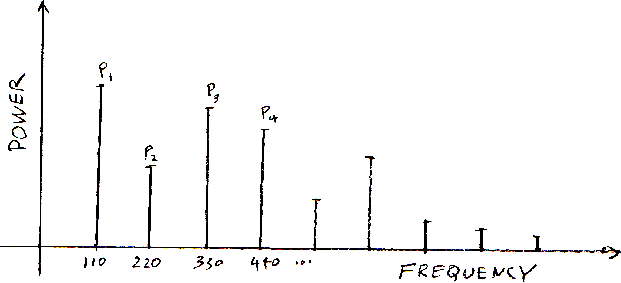Here the numbers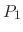, ..., represent the average power of the sinusoidal components. (Alternatively we could specify their peak amplitudes since the two are related by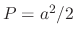. I chose power instead of amplitude to make clear the parallel between this and the following picture.)

Such a spectrum is called discrete because all the power is concentrated on a discrete set, that is, a set containing finite number of points per unit of frequency. The example here is furthermore a harmonic spectrum, meaning that the frequencies where there is power are all multiples of a fundamental frequency that is within the audible frequency range (in this case, 110 Hz). This is the spectrum of a complex periodic tone (section 2.2).

A discrete spectrum could also describe a complex inharmonic tone, in which case we say that the spectrum, too, is inharmonic.

## 3.1.2 Continuous Spectra

Signals or recordings that occur in nature never have discrete spectra; their spectra are continuous functions of frequency. A signal's continuous power spectrum might look as shown:Continuous power spectra can be (and perhaps usually are) measurements of a real signal over a finite period of time (for a signal) or a finite number of sample points (for a recording). A continuous power spectrum has a physical meaning: the area under the curve over a range of frequencies (say fromtois the total average power of the signal between those two frequencies. The area under the entire curve (from zero frequency to the highest possible one) is the total average power of the signal.

To put this another way: the continuous power spectrum describes how the average power of the signal is distributed over frequencies. Its units are power per frequency (for instance, watts per Hz.).

As an example, here is a segment of a sinusoid, that is, a sinusoid that is only computed for a finite amount of time. Although the true signal is a digital recording, it is labeled as if it were a signal depending on time; it has four cycles, at a frequency of one cycle per unit of time:Here is the signal's measured power spectrum; the horizontal axis is frequency in cycles per unit time and the vertical axis is power pre frequency, normalized so that the peak is one:There is a peak centered about a frequency of one, with width 1/2. There are other visible peaks, called sidelobes, which look a good bit smaller than the main, real" one. To see better what happened to our measurement, we relabel the vertical axis to show relative power in decibels, with the peak normalized to 100: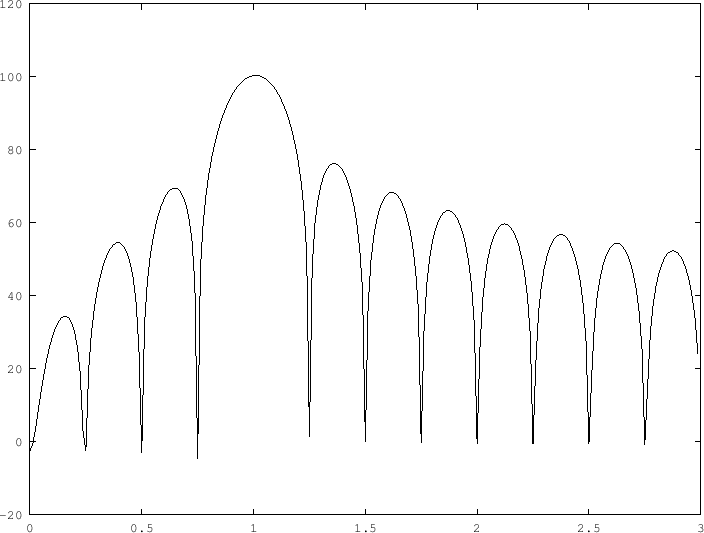We see that, if we don't have the luxury of waiting forever, our sinusoids can look very impure indeed. In general, if we only have access to a short segment of a signal (in this example, we had only four periods of a sinusoid), so that we have nothing better to suppose than that the signal is zero outside the time window we're looking at, our attempts to measure the signal's spectrum will give us only a blurry, out-of-focus result.

Why, then, don't we just collect a very long sample of the signal? Perhaps there is a practical reason we couldn't do that, but there's a deeper consideration: real signals that might arise in music, speech, or communications often change rapidly over time and in order to try to resolve how they are behaving in time we're obliged to examine them on short time scales. And the shorter the time scale we look on, more blurred in frequenct the spectrum will become. This limitation is well known in physics-- it's caller the Heisenberg Uncertainty Principle.

## 3.1.3 Short-time Spectra

Conceptually, the spectrum of a signal is an average over all of time. However, it is often desirable to find out what the spectrum of a signal is over a specific duration of time, or even to split a signal up into a sequence of short recordings and measure the spectra of each of these recordings separately. In this way, for a single input sound or recording, you would get a time-dependent spectrum.

To do this, for any timeyou would consider a small interval of time (say, fromtofor some fixed interval of time), and make up a new recording that consists only of those samples lying within the interval. (You can consider this extracted recording as being equal to zero outside the interval, exactly like the short sinusoidal burst we analyzed above.) and take the spectrum of that. The spectrum of this extracted signal is called a short-time spectrum. It depends on the choice of; the larger the segment you analyze the more sharply you can resolve frequencies but the less precisely you can resolve features in time.

# 3.2 Filters

Not only is a signal's spectrum a useful descriptive device, but it is one that we have some power to modify. Probably the most important tools we have for doing this are filters. A filter, for our purposes, is a process through which we can send any signal or recording, that multiplies the spectrum of the signal by a function of frequency known as the filter's frequency response. As a block diagram it might look like this:Ifis the filter's frequency response, and if you put in a sinusoid with amplitudeand frequency, the output of the filter will be a sinusoid with the same frequency but an amplitude of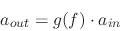The output might have a larger amplitude than the input at some frequencies and a smaller one at others, depending on whether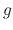is larger or smaller than one. The way we are defining gain implies that it is always zero or positive.

That implies that the power changes like this: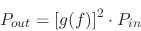and the level in decibels, like this:In a widely agreed-upon confusion of terminology, the gain in decibels, equal to, is often called the frequency response in decibels.

Of all the sorts of filters one could design, three specific types, low-pass, high-pass, and resonant filters, appear often. They have frequency responses as suggested here:Low-pass and high-pass filters are often used to get rid of, or at least decrease, the amplitude of high or low frequencies, respectively.

Resonant filters have at least two interesting functions. First, they can be used to imitate physical systems, such as cavities filled with air (your mouth or your ear canal, for example). A wah-wah guitar pedal is nothing but a foot-controlled resonant filter. Second, they permit us to (approximately) pick out a portion of a signal's spectrum in order to measure it (that's how one measures spectra in the first place) or in order to be able to treat different frequencies, that might be simultaneously present in a complex sound, in independently controllable ways.

A resonant filter typically has three parameters: a peak gain, a center frequency, and a bandwidth, as shown below: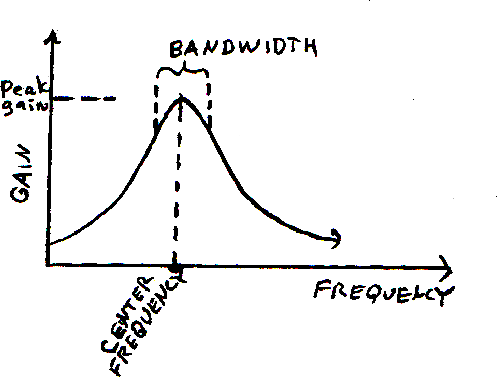The peak gain and center frequency have precise definitions (they are the two coordinates of the point at the apex of the curve). The bandwidth is a looser notion. One often-used measure is the distance between two points on the frequency axis where the curve is a specific relative amount (often 3 decibels) lower than the peak.

# 3.3 Application: Subtractive Synthesis

Real-world sounds frequently have time-varying timbres. The most prominent example of this is the human voice, in which (as we'll see in Chapter 5) the formation of vowels and consonants is reflected in variations in the voice's short-time spectrum, which can also be heard as changes in timbre. Other musical instruments behave in the same way; for instance, brass instruments tend to sound brighter when they are played louder, and it would be desirable to be able to make electronic instruments whose sounds can change in similar (or perhaps opposing) ways.

One excellent way to do this is to start with either noise or a complex sum of sinusoids (there are many ways to come by one of these, for instance simply by playing a short recording in a loop many times per second) and apply a filter whose properties vary appropriately with time. Here, for instance, is a simple sound to begin with:

SOUND EXAMPLE: Recording of a pulse, repeated 110 times per second; 5 second duration.

Here is its measured spectrum: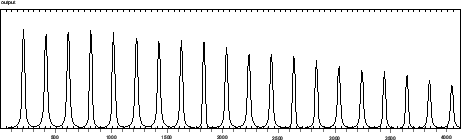If we send that recording through a resonant filter, with center frequency 880 and bandwidth about 300, we get a spectrum like this: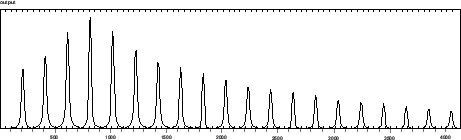The center frequency or bandwidth could be varied in time. In the following sound example we vary the center frequency from 110 to about 3000 and back:

SOUND EXAMPLE: subtractive synthesis in which the sound described above is put through a sweeping filter.

One powerful aspect of this particular technique is that one isn't limited to using very simple recordings as sources. To make just one example, white noise (which we've mentioned before but haven't been able to do very much with) is an excellent starting point for subtractive synthesis. Applying exactly the same sweeping filter before to a recording of white noise gives us this:

SOUND EXAMPLE: subtractive synthesis; same filter, white noise as input.

Here is the spectrum corresponding to the one above (880 Hz. center frequency):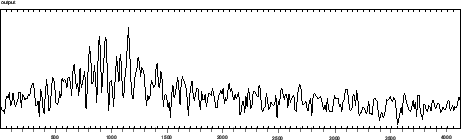# 3.4 The Inner Ear as Filterbank

Some limited insight about how timbre (which is a subjective quality of a sound) might relate to spectrum (a measurable property of a signal or recording) can be gained by studying how hearing works. Hearing is in general a ferociously complicated thing that humans are unlikely ever to understand, except in essentially trivial aspects. However, the things we do pretend to understand can often suggest interesting things to try in working with computer-mediated sound.

The active element of human hearing is the part of the human body that translates mechanical motion into nerve activation. This is a tiny, coiled, worm-shaped device in the inner ear called the cochlea. Vibrations that travel down its length get stronger and weaker as they travel in such a way that different frequencies are more prominent at different locations. It is a great simplification but not a complete misrepresentation to regard this as an array of resonant filters wired in parallel. Such an array is called a filterbank. Among other things, the ear seems to estimate the short-time spectrum of incoming sounds by measuring the average power, over short intervals of time, that shows up at various points along the cochlea.

Something is known about the frequency response of the filters" that predict cochlear vibrations up and down its length. As a very rough indication, the bandwidths are about 100 Hz. up to a center frequency of about 550 Hz (or, equivalently, up until the lower edge of the band is 500 Hz.) For center frequencies above 550 Hz. the bandwidth is about 20 percent of the frequency of the lower side of the band (or, equivalently, 18 percent of the center frequency). If you lay filters out side to side obeying these proportions, it takes about 24 of them to fill the frequency range of human hearing. This set of frequency ranges (often specified as the 24 intervals between the 25 frequencies 0, 100, 200, 300, 400, 510, 630, 770, 920, 1080, 1270, 1480, 1720, 2000, 2320, 2700, 3150, 3700, 4400, 5300, 6400, 7700, 9500, 12000, 15500) are known as the bark scale. So 100 Hz. is one bark, 1080 Hz. is 9 barks, and so on.

A range of frequencies corresponding to one location along the cochlea (from 100 to 200 Hz, say) is called a critical band. Critical bands overlap; for instance, 110 to 210 Hz. would also be considered one. The bandwidth of a critical band is always one bark.

Measuring the short-time power spectrum of a signal arranged in Barks can offer a rough visual idea of the overall loudness and timbre of a sound. In particular, the perceived strength of a signal within a critical band appears to depend on the total power within the band. This total power then has to be converted to perceived loudness (using a conversion unit called the sone) and then all the loudnesses are added (in sones) to get the resulting overall loudness.

# Exercises and Project

1. In a complex, periodic tone, how many harmonics lie between two and three octaves above the fundamental (not including the lower and upper limit)?

2. What is the interval, in half tones (twelfths of an octave), between the second and third harmonic of a complex harmonic tone?

3. A low-pass filter has a frequency-dependent gain ofWhat is the gain, in decibels, at 1000Hz?

4. If you send a sinusoid at frequency 100 Hz. and average power one, through the filter of exercise 3, what is the average power of the output?

5. What is the lowest-frequency pair of partials of a 1000-Hz.complex harmonic tone that lies within a critical band?

6. If two frequencies above 550 Hz. are separated by one bark, how many half-tones are they apart?

Project: Critical bands and loudness. This project tries to investigate how loudnesses of clusters of sinusoids are perceived differently when they are spaced withing a critical band than otherwise. For this experinent you should try to set yourself up with a reasonable listening environment, either using headphones or playing through a stereo (but not your laptop speaker).

Start by connecting a single sinusoid" object with frequency 1000 Hz. to an switch" object (these objects are both in the Music 170 library).

Now make another version (in the same patch) with four sinusoids tuned to 960, 980, 1000, anad 1020 Hz.. Connect all four to th input of a second output" so that you can turn them on and off as a group, independently of the first one.

Make a third group of objects in the same way (or just duplicate the second group) but now set the frequencies to 500. 1000, 2000, and 4000.

Now, by turning them on and off (using the onoff control on the three output objects) equalize the outputs until all three are at a comfortable (reasonably soft) listening level. (If you have to push any of the output gains past about 90 dB, you should turn up your speaker instead. On my system I'm using gain values between 50 and 70.)

Now adjust the three output gains so that, as you turn them on one at a time, you judge them to have roughly equal loudnesses. Write down the three gain values you had to use to equalize them.

Since the four frequencies are roughly at the same level on the equal-loudness contour chart (Wikipedia is your friend), the different frequencies should be less a factor than the spacing. Is it in fact nearly true (or totally false) that in the close spacing example, you ended up adjusting the comples tone so that its power was roughly equal to the power of the single 1000 Hz. tone? Is that still true when the four frequencies are spread widely (500-4000)?Next: 4. Pitches, Intervals, and Up: course-notes Previous: 2. Sinusoids   Index
msp 2014-11-24